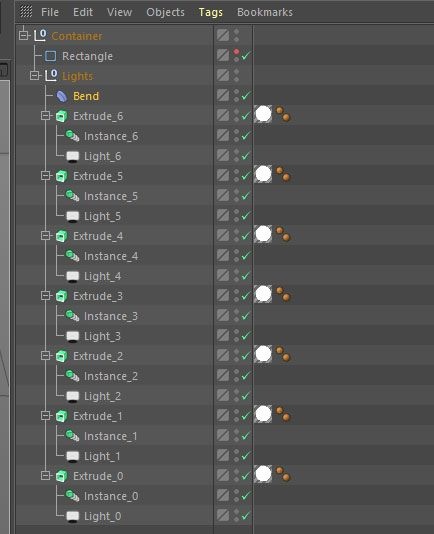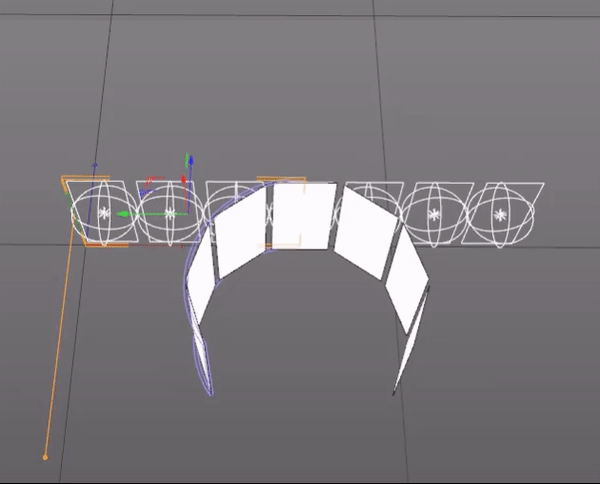# Bend Deformer Using C++

• Hello there, I would like to take this opportunity to wish you a very Happy New Year 2020.
I'm working on a project and I'm stuck on the following problem:
The Cinema 4D Bend Deformer object can be applied only on geometry, but I'm searching solution to create a similar deformation effect, that can be applied on a group of Light objects. I have tried a lot off methods and without any success.

Below you find a summary of the code that I'm currently using:

Code:

``````AutoAlloc<BaseObject>	containertPtr(Onull);
AutoAlloc<BaseObject>	parentlighstPtr(Onull);
AutoAlloc<BaseObject>	recPtr(Osplinerectangle);
AutoAlloc<BaseObject>	bendPtr(Obend);

if (!containertPtr || !recPtr || !parentPtr || !bendPtr)
return false;

// Set names
containertPtr->SetName("Container");
parentPtr->SetName("Lights");
recPtr->SetName("Rectangle");

// Variables
Int32 Count = 7;

Float	Xpos, Ypos,
Width = 150.0,
Height = 250.0,
interval = 40.0;

Ypos = Height / 2;

// Rectangle object
recPtr->SetParameter(PRIM_RECTANGLE_WIDTH, Width, DESCFLAGS_SET_0);
recPtr->SetParameter(PRIM_RECTANGLE_HEIGHT, Height, DESCFLAGS_SET_0);
recPtr.Release()->InsertUnder(containertPtr);

// Bend object
Float fullWidthSize = (Width*Count) + interval*(Count - 1);
Float	bendStrength = 90.0;

bendPtr->SetParameter(DEFORMOBJECT_SIZE, Vector(Height, fullWidthSize / 2, 10.0), DESCFLAGS_SET_0);
bendPtr->SetParameter(DEFORMOBJECT_MODE, DEFORMOBJECT_MODE_UNLIMITED, DESCFLAGS_SET_0);
bendPtr->SetParameter(DEFORMOBJECT_STRENGTH, bendStrength, DESCFLAGS_SET_0);
bendPtr->SetAbsPos(Vector(fullWidthSize / 4, Height / 2, 0.0));

for (Int32 i = 0; i < Count; i++)
{
AutoAlloc<BaseObject>	InstancePtr(Oinstance);
AutoAlloc<BaseObject>	ExtrudePtr(Oextrude);
AutoAlloc<BaseObject>	LightPtr(Olight);

if (!InstancePtr || !ExtrudePtr || !LightPtr)
return false;

Xpos = (Width*i) + (interval*i) + (Width / 2);

// Set names
ExtrudePtr->SetName("Extrude_" + String::IntToString(i));
InstancePtr->SetName("Instance_" + String::IntToString(i));
LightPtr->SetName("Light_" + String::IntToString(i));

// Light object
LightPtr->SetParameter(LIGHT_TYPE, 8, DESCFLAGS_SET_0);
LightPtr->SetAbsPos(Vector(Xpos, Ypos, 0));
LightPtr.Release()->InsertUnder(ExtrudePtr);

// Instance object
InstancePtr.Release()->InsertUnder(ExtrudePtr);

// Extrude object
ExtrudePtr->SetParameter(EXTRUDEOBJECT_MOVE, Vector(0, 0, 0), DESCFLAGS_SET_0);
ExtrudePtr->SetParameter(EXTRUDEOBJECT_HIERARCHIC, true, DESCFLAGS_SET_0);
ExtrudePtr->SetAbsPos(Vector(Xpos, Ypos, 0));
ExtrudePtr.Release()->InsertUnder(parentPtr);
}

bendPtr.Release()->InsertUnder(parentPtr);
parentPtr.Release()->InsertUnder(containertPtr);
...
``````

Screenshot of the generated scene:Preview of the bend deformation, when I manipulate the strength value of my the Bend object. You see that the bend deformer affect only the Extrude objects and not the Light objects:If you have any suggestion on the method that I can use to reproduce a similar deformation as an Bend Deformer using C++, but can be applied on group of Light objects. I would be most grateful.

Thank you.

• The only thing I can think of would be to Clone a light onto a Matrix and apply the bend to the Matrix object. The resulting positions and rotations of the Matrix should follow the bend but you will not get any deformation of the lights. They will still be flat lights but they should be distributed along the bend.

• @wuzelwazel
Hi, I tried your solution but it give same result, the Bend deformer don't affect the Light objects, all the lights objects remain unmoved. I can only play with the Step Rotation parameter of the Cloner object.

• If I understand the code correctly, you are only using it for generating the structure, and you rely on the Bend deformer completely to create the repositioning.

What if you lose the bend deformer, and use a Python tag instead that creates the bend effect "manually" - based on not the polygons (which you don't deform in your screenshot anyway) but on the object matrix of the affected objects, which would then apply to the lights as well? Is that wavy motion in the screenshot all you want to generate, or is there more to it?

• @Cairyn
Yes, the current code is only to generate the structure. in an another test I tried to create an algorithm to moving and rotate individually the lights objects inside the loop, and I realized that is too complex for me to reproduce the same bend deformation. so, I gave up the idea.

I did not say it, but I'm using this inside GetVirtualObjects, I don't know if is allowed to add Python tag inside GVO.
and then I will control the bend deformation from the via my GVO object Description Resource.

Yes, I'm just searching how to animate the lights objects same as the Extrude object in the second screenshot.

• @mfersaoui If you're generating the objects on the fly, you wouldn't need a Python tag but could place the objects in their "bent" placement already, so that would not be a problem. Of course, if you can't replicate the bending algorithm the question is moot... but maybe Google knows the fitting formula?

• Hi,

while Cinema's restriction of deformers to point data is certainly a shortcoming, I do not understand (because I might be overlooking something) why you do not write a bend function yourself.

All the common deformers are just translation, rotation or scale operations modified by a linear interpolation along an axis of operation. The bend operation is just a rotation operation where the rotation angle is interpolated along the axis of operation.

Cheers
zipit

• @zipit
Hi,
As I explained to @Cairyn I tried to create an algorithm to do that, but this is too complex for me and I haven't level to do that. I will search again in google maybe I will find something similar.

• @zipit @mfersaoui not quite; the pivot of the rotation is also changing (only along one axis, but still...), and it's not a linear movement but some exponential one between 0 (approaching only) and infinity (when no bend). The infinity one may also be tricky numerically.

I have an idea how to solve (my Google-fu seems weak on this) but haven't tried in praxis yet.

• okay, calculate with me...

The arc length L for an angle alpha on a circle with radius r is L = 2* pi * r * alpha / 360
(assuming alpha is in degree). alpha is known; it is the bend angle.
The arc length is also known - it's the distance of the bending point to the center of the bend
(assuming we want to keep that length constant).
The radius is actually the wanted value. So we transform
L * 360 = 2 * pi * r * alpha
(L * 360) / (2 * pi * alpha) = r
Obviously, this is numerically bad since with alpha approaching 0, r is approaching infinity. But maybe we can solve this in the transformation and get rid of either alpha or r.

The rotation of the target coordinate system (the object to place on the bend) is obviously alpha itself.
The position p of the target needs to be moved according to sin and cos of alpha on the circle with radius r.

Remember that all of this needs to be expressed in the coordinate systems of either the object, the world, or the bend deformer, which makes the calculation perhaps a bit awkward.

• For simplification, we'll assume that world, bend, and object coordinates are all the same.

Now you can draw a circle with radius r and check where a point p is going. Let's just look at the point p = (L,0) - the farthest centered point of our object, or the center of the coordinate system of out object if you want to move a whole object.

Then p'.x is going to be r * sin(alpha), and p'.z will be r - r * cos(alpha), as per the definitions of sine and cosine.

And that's all there is! Okay, after you did the necessary coordinate transformations between the base coordinate systems and adapted the calculation for other points than p, but that's homework.

Here I made a little scene where a Python tag does the calculation. A flattened cube is bent by a bend deformer; a torus primitive is moved accordingly to stay flush with the left edge of the cube. Note that the calculation for the torus position and rotation is done solely through the Python tag, whose only input the bend deformer's angle is, so the Python tag is actually replicating the bend deformer functionality.

20200104_CenterOfBendDeformer.c4d

The main part is the code of the Python tag (I'm calculating with radians here so don't wonder why there is no pi; also the bend deformer in my scene is pointing to the negative x so we have additional minus signs:

``````import c4d
from math import sin, cos

def main():
torus = op.GetObject()
source = torus.GetPred().GetDown()
strength = source[c4d.DEFORMOBJECT_STRENGTH]
originx = -400.0
originz = 0.0
if strength == 0.0:
targetx = originx
targetz = originz
roth = 0.0
else:

alpha = strength

roth = -alpha

torus[c4d.ID_BASEOBJECT_REL_POSITION,c4d.VECTOR_X] = targetx
torus[c4d.ID_BASEOBJECT_REL_POSITION,c4d.VECTOR_Z] = targetz
torus[c4d.ID_BASEOBJECT_REL_ROTATION,c4d.VECTOR_X] = roth
``````

Obviously this is a very simplified sample that works only for this scene, but you can adapt the calculation for yours.

• @Cairyn
Hi,
Thank you so much for your help and for your detailed explanation, I started to testing your code in python to better understand and now I will try to adapt it to my project in c++. If I have a problem I return to this topic.
Thanks again.

• @Cairyn
Thanks to you, I have obtained the desired result.
In my project the "strength" and the "originx" values is not constant, also the total count of the lights object is not constant. So I made the necessary changes on your code.
Here is the final code:

``````...

AutoAlloc<BaseObject>	containertPtr(Onull);
AutoAlloc<BaseObject>	parentlighstPtr(Onull);
AutoAlloc<BaseObject>	recPtr(Osplinerectangle);
AutoAlloc<BaseObject>	bendPtr(Obend);

if (!containertPtr || !recPtr || !parentPtr || !bendPtr)
return false;

// Set names
containertPtr->SetName("Container");
parentPtr->SetName("Lights");
recPtr->SetName("Rectangle");

// Variables
Int32 Count = 7;

Float	Xpos, Ypos,
Width = 150.0,
Height = 250.0,
interval = 40.0;

Ypos = Height / 2;

// Rectangle object
recPtr->SetParameter(PRIM_RECTANGLE_WIDTH, Width, DESCFLAGS_SET_0);
recPtr->SetParameter(PRIM_RECTANGLE_HEIGHT, Height, DESCFLAGS_SET_0);
recPtr.Release()->InsertUnder(containertPtr);

// Bend object
Float fullWidthSize = (Width*Count) + interval*(Count - 1);
Float	bendStrength = 90.0;

bendPtr->SetParameter(DEFORMOBJECT_SIZE, Vector(Height, fullWidthSize / 2, 10.0), DESCFLAGS_SET_0);
bendPtr->SetParameter(DEFORMOBJECT_MODE, DEFORMOBJECT_MODE_UNLIMITED, DESCFLAGS_SET_0);
bendPtr->SetParameter(DEFORMOBJECT_STRENGTH, bendStrength, DESCFLAGS_SET_0);
bendPtr->SetAbsPos(Vector(fullWidthSize / 4, Height / 2, 0.0));

Float strength, alpha, radius, originx, originz = 0.0, targetx, targetz, roth, step;

Float	centerx = fullWidthSize/2;

for (Int32 i = 0; i < Count; i++)
{
AutoAlloc<BaseObject>	InstancePtr(Oinstance);
AutoAlloc<BaseObject>	ExtrudePtr(Oextrude);
AutoAlloc<BaseObject>	LightPtr(Olight);

if (!InstancePtr || !ExtrudePtr || !LightPtr)
return false;

Xpos = (Width*i) + (interval*i) + (Width / 2);

originx = centerx - Xpos;
originx = originx*-1;

step = centerx / originx;
strength = bendStrength / step;

if (strength == 0.0) {
targetx = originx;
targetz = originz;
roth = 0.0;
}
else {
alpha = strength;

roth = -alpha;
}
// Set names
ExtrudePtr->SetName("Extrude_" + String::IntToString(i));
InstancePtr->SetName("Instance_" + String::IntToString(i));
LightPtr->SetName("Light_" + String::IntToString(i));

// Light object
LightPtr->SetParameter(LIGHT_TYPE, 8, DESCFLAGS_SET_0);
LightPtr.Release()->InsertUnder(ExtrudePtr);

// Instance object
InstancePtr.Release()->InsertUnder(ExtrudePtr);

// Extrude object
ExtrudePtr->SetParameter(EXTRUDEOBJECT_MOVE, Vector(0, 0, 0), DESCFLAGS_SET_0);
ExtrudePtr->SetParameter(EXTRUDEOBJECT_HIERARCHIC, true, DESCFLAGS_SET_0);

ExtrudePtr->SetAbsPos(Vector(targetx, Ypos, targetz));
ExtrudePtr->SetAbsRot(Vector(roth, 0, 0));

ExtrudePtr.Release()->InsertUnder(parentPtr);
}

bendPtr.Release()->InsertUnder(parentPtr);
parentPtr.Release()->InsertUnder(containertPtr);
...
``````

• I'm not sure why my suggested solution didn't work. Did you apply the Bend to a Matrix object and then Clone onto that Matrix (Cloner in Object mode targeting the Matrix)? The Bend should modify the Matrix positions/rotations and the Cloner should pick up those modified positions for it's children (the lights).

Rolling your own bend algorithm definitely has its advantages over this, but I was surprised to hear that you got exactly the same result.

• @wuzelwazel
Hi,

My bad, I misunderstood. I just retest and it's working well using Matrix and Cloner object.
Thanks you so much for your help and for reminding me.

• Not that much to add it here I think everything was already said.

But I just would like to remind you to use the Q&A functionality
Cheers,
Maxime.